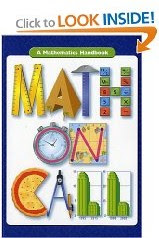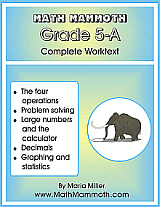## Posts

Showing posts from August, 2009

### Math trick and its proof: square a number ending in 5I will be hosting the blog carnival Math Teachers at Play next week. (You can send in submissions here .) One submission I got about various multiplication tricks or shortcuts got me inspired to write a proof of the particular trick. You could definitely use this in algebra class. First explain the shortcut or trick itself. Then ask students to prove it, or to explain WHY it works, using algebra. You could also explain this to younger students as an additional "neat trick" and let them explore and play with it. THE "TRICK" If a number ends in 5, then its square can be calculated using this "trick" (I like to call it a shortcut because there's nothing magic about it): Let's say we have 75 × 75 => Go 7 × 8 = 56. Then tag 25 (or 5 × 5) into that. You get 5625. Let's say we have 35 × 35 => Go 3 × 4 = 12. Then tag 25 into that. You get 1225. Let's say we have 115 × 115 => Go 11 × 12 = 132. Then tag 25 into that. You get 13,2

### A math review or reference book for adults & studentsI just stumbled upon the book Math on Call: A Mathematics Handbook : Based on the reviews and from the "Look Inside" feature at Amazon it looks like a GREAT math reference book . I've been on the lookout for something like this, because I get emails from adults who want to review or refresh their basic math before going to college or some other course they're taking. So now I have something to refer them to. The table of contents includes these chapters: NUMERATION The Base Ten System Rational Numbers Irrational Numbers NUMBER THEORY Factors and Multiples Powers and Roots COMPUTATION Addition Subtraction Multiplication Division ALGEBRA Algebraic Notation Properties Relations and Functions Equations Inequalities GRAPHS AND STATISTICS Gathering Data Organizing and Summarizing Data with Statistics Displaying Data in Tables and Graphs Interpreting Data GEOMETRY Elements of Geometric Figures Angles Plane Figures Solid Figures RATIO, PROPORTION, AND

### Math Teachers at Play Carnival

There is lots of interesting stuff today at Math Teachers at Play Carnival #14 at Math Mama Writes. Quite a nice assortment - surely something for everyone! I particularly recommend Three Applications of High, Abstract Math . We teachers can always use other ideas as to how mathematics is useful in real life.

### Learn to recognize coinsImage by Stopnlook In the past few days I decided it's time for my younger girl to start learning how to recognize coins and learn the cent-values of various coins. She's generally been doing kindergarten-1st grade addition and subtraction and I figured she's ready. First, I got a pile of pennies, a pile of nickels, and a pile of dimes (no quarters at first). We played where I told her to make a certain amount, such as "Make 24 cents." She made it, I checked. Then she told me to make some amount. We just take turns. It's like a game, and great fun for her! I figure it'll work the same with other kindergarten or 1st grade kids. Since she did pretty good, I was able to introduce quarters the next day. I showed her that two of them makes 50, and we practiced making 62 or 58 or other such amounts that use 2 quarters. Then I showed her a quarter and a nickel, and we figured out how much that was. That's always a difficult thing - to combine just one q

### Review of Algebra Unplugged

This review has been moved here .

### Multiplication Mountain songs CD - a review

The review of Multiplication Mountain has been moved here .

### Math Mammoth Grade 5 Complete Curriculum now available163 pages 147 lesson pages 218 pages 179 lesson pages 5-A contents and samples 5-B contents and samples Finally it is ready! I know some folks have been waiting for the 5-B part to get finished, and now it is here! Math Mammoth Grade 5 complete curriculum consists of two student worktexts (A and B), a separate answer key for each, chapter tests and an end-of-year test, cumulative reviews, and an easy worksheet maker (Internet access required) to make extra practice worksheets when needed. The two books (worktext part A and part B) for 5th grade deal with multi-digit multiplication and long division simple equations problem solving place value with large numbers and the judicious use of calculator all operations with decimals statistics and graphing all fraction operations geometry: classifying and drawing triangles & quadrilaterals; calculating the area of rectangles, triangles, parallelograms, and compound figures; surface area and volume of rectangular prisms introduct